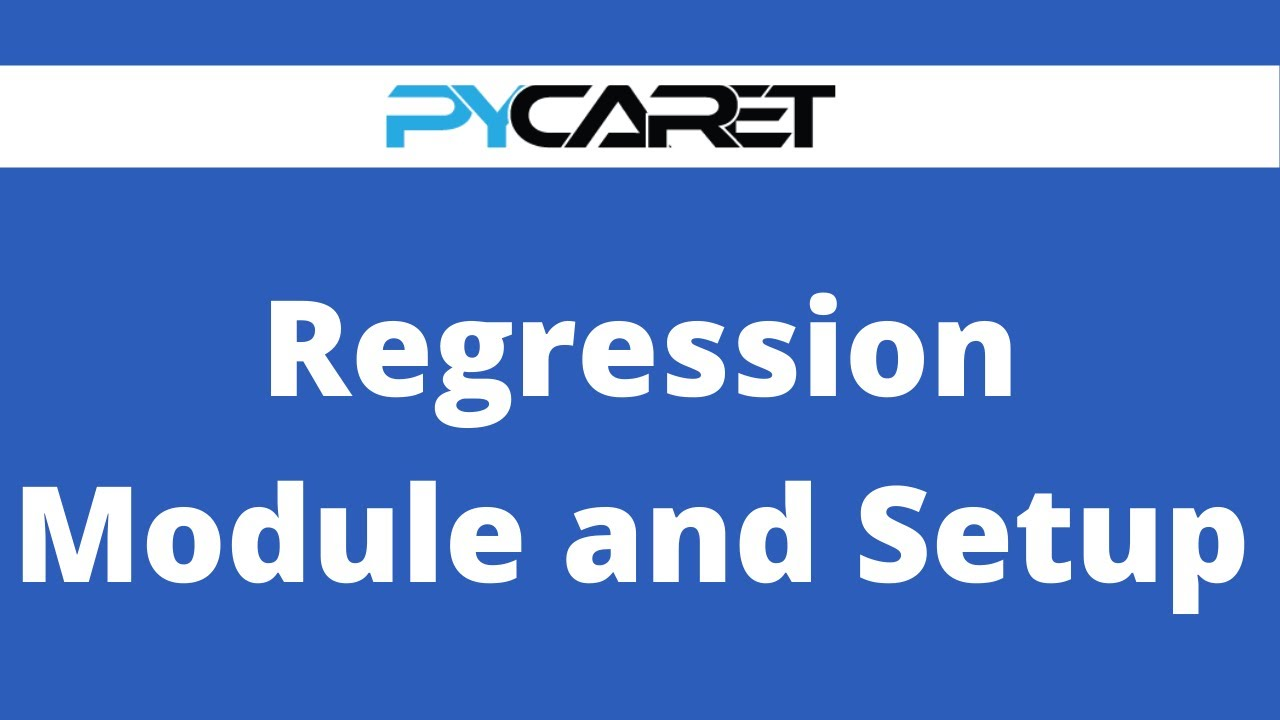1611286074

# Regression Module and Setting Up Environment in PyCaret

In this video, I will show you how to import regression module and set up the environment

#pycaret #python #machine-learning

## Buddha Community1611286074

## Regression Module and Setting Up Environment in PyCaret

In this video, I will show you how to import regression module and set up the environment

#pycaret #python #machine-learning1592023980

## 5 Regression algorithms: Explanation & Implementation in Python

Take your current understanding and skills on machine learning algorithms to the next level with this article. What is regression analysis in simple words? How is it applied in practice for real-world problems? And what is the possible snippet of codes in Python you can use for implementation regression algorithms for various objectives? Let’s forget about boring learning stuff and talk about science and the way it works.

#linear-regression-python #linear-regression #multivariate-regression #regression #python-programming1598352300

## Regression: Linear Regression

Machine learning algorithms are not your regular algorithms that we may be used to because they are often described by a combination of some complex statistics and mathematics. Since it is very important to understand the background of any algorithm you want to implement, this could pose a challenge to people with a non-mathematical background as the maths can sap your motivation by slowing you down.In this article, we would be discussing linear and logistic regression and some regression techniques assuming we all have heard or even learnt about the Linear model in Mathematics class at high school. Hopefully, at the end of the article, the concept would be clearer.

**Regression Analysis **is a statistical process for estimating the relationships between the dependent variables (say Y) and one or more independent variables or predictors (X). It explains the changes in the dependent variables with respect to changes in select predictors. Some major uses for regression analysis are in determining the strength of predictors, forecasting an effect, and trend forecasting. It finds the significant relationship between variables and the impact of predictors on dependent variables. In regression, we fit a curve/line (regression/best fit line) to the data points, such that the differences between the distances of data points from the curve/line are minimized.

#regression #machine-learning #beginner #logistic-regression #linear-regression #deep learning1611383425

## Clustering Module and Setting Up Environment in PyCaret

In this video, I will show you how to import clustering module and set up the environment

#pycaret #python1611561540

## Anomaly Detection Module and Setting Up Environment in PyCaret

In this video, We will show you how to import anomaly detection module and set up the environment

#pycaret #python